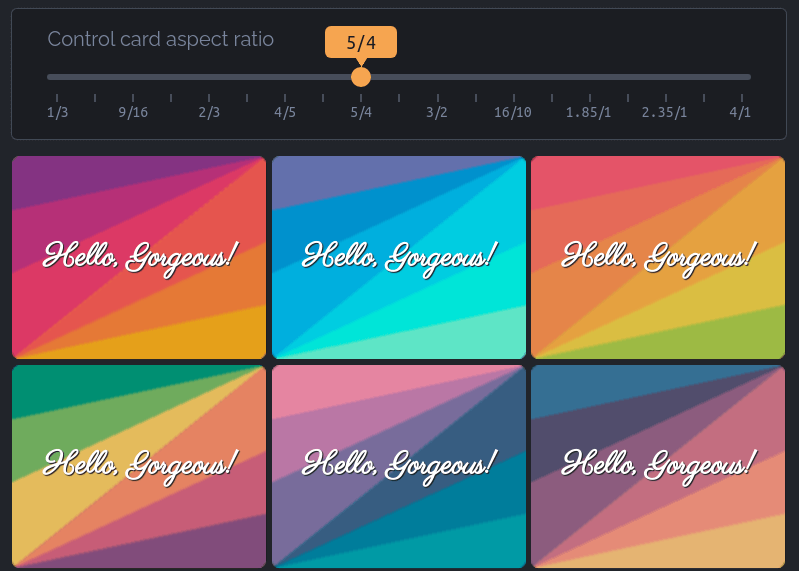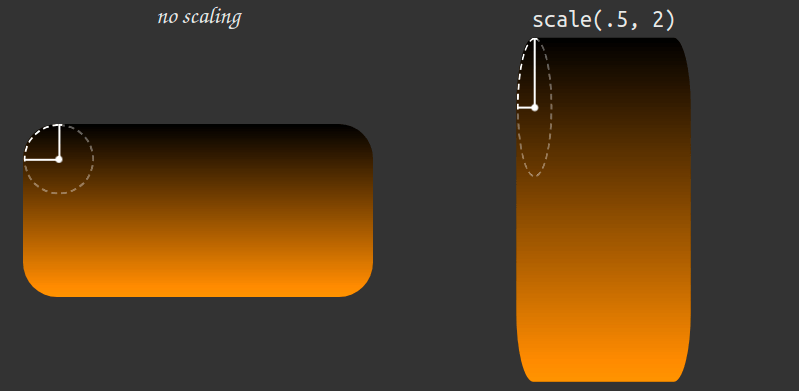Strategy

# Variable Aspect Ratio Card With Conic Gradients Meeting Alon…

I recently came across an interesting problem. I had to implement a grid of cards with a variable (user-set) aspect ratio that was stored in a `--ratio` custom property. Boxes with a certain aspect ratio are a classic problem in CSS and one that got easier to solve in recent years, especially since we got `aspect-ratio`, but the tricky part here was that each of the cards needed to have two conic gradients at opposite corners meeting along the diagonal. Something like this:User set aspect ratio cards.

The challenge here is that, while it’s easy to make an abrupt change in a `linear-gradient()` along the diagonal of a variable aspect ratio box using for example a direction like `to top left` which changes with the aspect ratio, a `conic-gradient()` needs either an angle or a percentage representing how far it has gone around a full circle.

Check out this guide for a refresher on how conic gradients work.

### The simple solution

The spec now includes trigonometric and inverse trigonometric functions, which could help us here — the angle of the diagonal with the vertical is the arctangent of the aspect ratio `atan(var(--ratio))` (the left and top edges of the rectangle and the diagonal form a right triangle where the tangent of the angle formed by the diagonal with the vertical is the width over the height — precisely our aspect ratio).The angle of the diagonal with the vertical (edge).

Putting it into code, we have:

``````--ratio: 3/ 2;
aspect-ratio: var(--ratio);
--angle: atan(var(--ratio));
background:
/* below the diagonal */
conic-gradient(from var(--angle) at 0 100%,
#319197, #ff7a18, #af002d  calc(90deg - var(--angle)), transparent 0%),
/* above the diagonal */
conic-gradient(from calc(.5turn + var(--angle)) at 100% 0,
#ff7a18, #af002d, #319197 calc(90deg - var(--angle)));``````

However, no browser currently implements trigonometric and inverse trigonometric functions, so the simple solution is just a future one and not one that would actually work anywhere today.

### The JavaScript solution

We can of course compute the `--angle` in the JavaScript from the `--ratio` value.

``````let angle = Math.atan(1/ratio.split("https://css-tricks.com/").map(c => +c.trim()).reduce((a, c) => c/a, 1));
document.body.style.setProperty('--angle', `\${+(180*angle/Math.PI).toFixed(2)}deg`)``````

But what if using JavaScript won’t do? What if we really need a pure CSS solution? Well, it’s a bit hacky, but it can be done!

### The hacky CSS solution

This is an idea I got from a peculiarity of SVG gradients that I honestly found very frustrating when I first encountered.

Let’s say we have a gradient with a sharp transition at `50%` going from bottom to top since in CSS, that’s a gradient at a `0°` angle. Now let’s say we have the same gradient in SVG and we change the angle of both gradients to the same value.

In CSS, that’s:

``linear-gradient(45deg, var(--stop-list));``

In SVG, we have:

``````<linearGradient id='g' y1='100%' x2='0%' y2='0%'
gradientTransform='rotate(45 .5 .5)'>
<!-- the gradient stops -->
</linearGradient>``````

As it can be seen below, these two don’t give us the same result. While the CSS gradient really is at `45°`, the SVG gradient rotated by the same `45°` has that sharp transition between orange and red along the diagonal, even though our box isn’t square, so the diagonal isn’t at `45°`!45° CSS vs. SVG gradient (live demo).

This is because our SVG gradient gets drawn within a `1x1` square box, rotated by `45°`, which puts the abrupt change from orange to red along the square diagonal. Then this square is stretched to fit the rectangle, which basically changes the diagonal angle.

Note that this SVG gradient distortion happens only if we don’t change the `gradientUnits` attribute of the `linearGradient` from its default value of `objectBoundingBox` to `userSpaceOnUse`.

#### Basic idea

We cannot use SVG here since it only has linear and radial gradients, but not conic ones. However, we can put our CSS conic gradients in a square box and use the `45°` angle to make them meet along the diagonal:

``````aspect-ratio: 1/ 1;
width: 19em;
background:
/* below the diagonal */
conic-gradient(from 45deg at 0 100%,
#319197, #ff7a18, #af002d 45deg, transparent 0%),
/* above the diagonal */
conic-gradient(from calc(.5turn + 45deg) at 100% 0,
#ff7a18, #af002d, #319197 45deg);``````

Then we can stretch this square box using a scaling `transform` – the trick is that the ‘/’ in the `3/ 2` is a separator when used as an `aspect-ratio` value, but gets parsed as division inside a `calc()`:

``````--ratio: 3/ 2;
transform: scaley(calc(1/(var(--ratio))));``````

You can play with changing the value of `--ratio` in the editable code embed below to see that, this way, the two conic gradients always meet along the diagonal:

Note that this demo will only work in a browser that supports `aspect-ratio`. This property is supported out of the box in Chrome 88+ (current version is 90), but Firefox still needs the `layout.css.aspect-ratio.enabled` flag to be set to `true` in about:config. And if you’re using Safari… well, I’m sorry!Enabling the flag in Firefox.

#### Issues with this approach and how to get around them

Scaling the actual `.card` element would rarely be a good idea though. For my use case, the cards are on a grid and setting a directional scale on them messes up the layout (the grid cells are still square, even though we’ve scaled the `.card` elements in them). They also have text content which gets weirdly stretched by the `scaley()` function.

The solution is to give the actual cards the desired `aspect-ratio` and use an absolutely positioned `::before` placed behind the text content (using `z-index: -1`) in order to create our `background`. This pseudo-element gets the `width` of its `.card` parent and is initially square. We also set the directional scaling and conic gradients from earlier on it. Note that since our absolutely positioned `::before` is top-aligned with the top edge of its `.card` parent, we should also scale it relative to this edge as well (the `transform-origin` needs to have a value of `0` along the y axis, while the x axis value doesn’t matter and can be anything).

``````body {
--ratio: 3/ 2;
/* other layout and prettifying styles */
}

.card {
position: relative;
aspect-ratio: var(--ratio);

&::before {
position: absolute;
z-index: -1; /* place it behind text content */

aspect-ratio: 1/ 1; /* make card square */
width: 100%;

/* make it scale relative to the top edge it's aligned to */
transform-origin: 0 0;
/* give it desired aspect ratio with transforms */
transform: scaley(calc(1/(var(--ratio))));
/* set background */
background:
/* below the diagonal */
conic-gradient(from 45deg at 0 100%,
#319197, #af002d, #ff7a18 45deg, transparent 0%),
/* above the diagonal */
conic-gradient(from calc(.5turn + 45deg) at 100% 0,
#ff7a18, #af002d, #319197 45deg);
content: '';
}
}``````

Note that we’ve moved from CSS to SCSS in this example.

This is much better, as it can be seen in the embed below, which is also editable so you can play with the `--ratio` and see how everything adapts nicely as you change its value.

#### Padding problems

Since we haven’t set a `padding` on the card, the text may go all the way to the edge and even slightly out of bounds given it’s a bit slanted.Lack of padding causing problems.

That shouldn’t be too difficult to fix, right? We just add a `padding`, right? Well, when we do that, we discover the layout breaks!Adding a padding breaks the layout. (Demo)

This is because the `aspect-ratio` we’ve set on our `.card` elements is that of the `.card` box specified by `box-sizing`. Since we haven’t explicitly set any `box-sizing` value, its current value is the default one, `content-box`. Adding a `padding` of the same value around this box gives us a `padding-box` of a different aspect ratio that doesn’t coincide with that of its `::before` pseudo-element anymore.

In order to better understand this, let’s say our `aspect-ratio` is `4/ 1` and the width of the `content-box` is `16rem` (`256px`). This means the height of the `content-box` is a quarter of this width, which computes to `4rem` (`64px`). So the `content-box` is a `16rem×4rem` (`256px×64px`) rectangle.

Now let’s say we add a `padding` of `1rem` (`16px`) along every edge. The width of the `padding-box` is therefore `18rem` (`288px`, as it can be seen in the animated GIF above) — computed as the width of the `content-box`, which is `16rem` (`256px`) plus `1rem` (`16px`) on the left and `1rem` on the right from the `padding`. Similarly, the height of the `padding-box` is `6rem` (`96px`) — computed as the height of the `content-box`, which is `4rem` (`64px`), plus `1rem` (`16px`) at the top and `1rem` at the bottom from the `padding`).

This means the `padding-box` is a `18rem×6rem` (`288px×96px`) rectangle and, since `18 = 3⋅6`, it has a `3/ 1` aspect ratio which is different from the `4/ 1` value we’ve set for the `aspect-ratio` property! At the same time, the `::before` pseudo-element has a width equal to that of its parent’s `padding-box` (which we’ve computed to be `18rem` or `288px`) and its aspect ratio (set by scaling) is still `4/ 1`, so its visual height computes to `4.5rem` (`72px`). This explains why the `background` created with this pseudo — scaled down vertically to a `18rem×4.5rem` (`288px×72px`) rectangle — is now shorter than the actual card — a `18rem×6rem` (`288px×96px`) rectangle now with the `padding`.

So, it looks like the solution is pretty straightforward — we need to set `box-sizing` to `border-box` to fix our problem as this applied the `aspect-ratio` on this box (identical to the `padding-box` when we don’t have a `border`).

Sure enough, this fixes things… but only in Firefox!Showing the difference between Chromium (top) and Firefox (bottom).

The text should be middle-aligned vertically as we’ve given our `.card` elements a grid layout and set `place-content: center` on them. However, this doesn’t happen in Chromium browsers and it becomes a bit more obvious why when we take out this last declaration — somehow, the cell in the card’s grid gets the `3/ 1` aspect ratio too and overflows the card’s `content-box`:Checking the card’s grid with vs. without place-content: center.

Fortunately, this is a known Chromium bug that should probably get fixed in the coming months.

In the meantime, what we can do to get around this is remove the `box-sizing`, `padding` and `place-content` declarations from the `.card` element, move the text in a child element (or in the `::after` pseudo if it’s just a one-liner and we’re lazy, though an actual child is the better idea if we want the text to stay selectable) and make that a `grid` with a `padding`.

``````.card {
/* same as before,
minus the box-sizing, place-content and padding declarations
the last two of which which we move on the child element */

&__content {
place-content: center;
padding: 1em
}
}``````

#### Rounded corners

Let’s say we also want our cards to have rounded corners. Since a directional `transform` like the `scaley` on the `::before` pseudo-element that creates our `background` also distorts corner rounding, it results that the simplest way to achieve this is to set a `border-radius` on the actual `.card` element and cut out everything outside that rounding with `overflow: hidden`.Non-uniform scaling distorts corner rounding. (Demo)

However, this becomes problematic if at some point we want some other descendant of our `.card` to be visible outside of it. So, what we’re going to do is set the `border-radius` directly on the `::before` pseudo that creates the card `background` and reverse the directional scaling `transform` along the y axis on the y component of this `border-radius`:

``````\$r: .5rem;

.card {
/* same as before */

&::before {
border-radius: #{\$r}/ calc(#{\$r}*var(--ratio));
transform: scaley(calc(1/(var(--ratio))));
/* same as before */
}
}``````

### Final result

Putting it all together, here’s an interactive demo that allows changing the aspect ratio by dragging a slider – every time the slider value changes, the `--ratio` variable is updated: